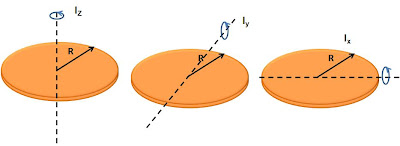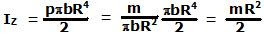## Tuesday, November 11, 2008

### Irodov Problem 1.240

Essentially in this problem Irodov asks you to prove the famous perpendicular axis theorem for moment of inertia. The proof is quite simple and given in the same location.From perpendicular axis theorem we know that Iz = Ix + Iy. Also since its a disc, the moment of inertia should not depend on which diameter is chosen, in other words Ix = Iy. Hence,
Ix = Iy = Iz/2.

In problem 1.239a I already found the moment of inertia Iz of a disc given by,Thus we have,